Principles of Population Genetics
Various aspects of "Population"

gene pool (a genetic unit):
all alleles at a (single) locus in a:
deme (an ecological unit):
all conspecific individuals in an area
(areas may be more or less defined)

panmictic unit (a reproductive unit):
group of interbreeding individuals
(interbreeding may be more or less random)

sample (a statistical unit):
subset of size 'N'
(population of N individuals has 2N alleles)

Theory of allele frequencies: minding  p's & q's

Genetic variation in populations described by genotype & allele frequencies
(not "gene" frequencies) [NS 01-01]

Consider a diploid autosomal locus with two alleles & no dominance
(=> semi-dominance: AA , Aa , aa  phenotypes distinguishable)

# AA =    # Aa = y    # aa = z    x + y + z = N (sample size)

f(AA) = x / N       f(Aa) = y / N       f(aa) = z / N

f(A) = (2x + y) / 2N          f(a) = (2z + y) / 2N

or    f(A) = f(AA) + 1/2 f(Aa)      f(a) = f(aa) + 1/2 f(Aa)

let p = f(A), q = f(a)    p & q are allele frequencies

Properties of p & q

p + q = 1     p = 1 - q    q = 1 - p

(p + q)=  p2 + 2pq + q2  =  1

(1 - q)2 + 2(1 - q)(q) + q2 = 1

p & q interchangeable wrt [read, "with respect to"] A & a

q usually used for
rarer, recessive, deleterious (disadvantageous), or "interesting" allele

BUT   'common' & 'rare' are statistical properties
'dominant' & 'recessive' are genotypic properties
'advantageous' & 'deleterious' are phenotypic properties
*** any combination of these properties is possible ***

The Hardy-Weinberg Theorem

What happens to p & q in one generation of random mating?

Consider a population of monoecious organisms that reproduce by random union of gametes

("tide pool" model)

(1) Determine expectation
of parental alleles coming together in various genotype combinations
expectation: the anticipated value of a variable
not quite the same as probability [NS 03-Box1]

Proofs by probability, binomial expansion, & Punnet Square methods [SR2019 3.1]
all show that expectation of f(AA) = p2
expectation of f(Aa) = 2pq
expectation of f(aa) = q2

(2) Re-describe offspring allele frequencies f(A') & f(a')

f(A') = f(AA) + 1/2 f(Aa)
= p2 + (1/2)(2pq) = p2 + pq = (p)(p+q) = p' = p

f(a') = f(aa) + 1/2 f(Aa)
= q2 + (1/2)(2pq) = q2 + pq = (q)(p+q) = q' = q

Hardy - Weinberg Theorem (1908):
Absent other genetic or evolutionary factors,
allele frequencies are invariant between generations,
& constant genotype frequencies reached in one generation.

p2 : 2pq : q2 are Hardy-Weinberg expectations / proportions (cf. Mendelian ratios 1 : 2 : 1 )

Not HW "equilibrium": frequencies change within & between generations during evolution

Hardy-Weinberg Expectations (HWE) obtained under more realistic conditions

(1) multiple alleles / locus

p + q + r = 1
(p + q + r)2 = p2 + 2pq + q2 + 2qr + r2 + 2pr = 1

Proportion of heterozygotes (H = 'heterozygosity')
measures genetic variation at a locus

Hobs = f(Aa) = observed heterozygosity
Hexp = 2pq   = expected heterozygosity (for two alleles)

He = 2pq + 2pr + 2qr = 1 - (p2 + q2 + r2)    for three alleles

n
He = 1 -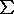(qi)2      for n alleles
i=1

where qi = freq. of i th allele of n alleles at a locus

Ex.: if q1 = 0.5, q2 = 0.3, & q3 = 0.2
then He = 1 - (0.52 + 0.32 + 0.22) = 0.62

*** HOMEWORK:

Calculate He 1) if q1 = 0.4, q2 = 0.3, q3 = 0.2, & q4 = 0.1
2) for a locus with 10 or 100 alleles, all at equal frequency

3) with one allele at q = 0.5 & 10 or 100 at equal frequency [note change]

iff [read: "if and only if"] allele frequencies in males & females equal
If frequencies initially unequal, they converge over several generations.

(3) dioecious organisms
sexes separate
HWE produced by random mating of individuals
expand (p2 'AA' + 2pq 'AB' + q2 'BB')2 :
nine possible mating types among genotypes
selfing (self-fertilization) remains possible

Application of Hardy-Weinberg Expectations (HWE) to evolutionary genetics

Genotype proportions in natural populations can be tested for HWE
Ho (null hypothesis): no other phenomena acting
NoteHWE often called a HW equilibrium, BUT
HWE observed only at birth of any single generation
changes bx newborns & adults
due to other factors:
=> HWE not an "equilibrium"
See Excel spreadsheets for Chi-Square calculations

Among Euro-Americans:
 MM MN NN Sum 1787 3039 1303 6129

f(M) = [(2)(1787) + 3039] / (2)(6129) = 0.539

f(N) = [(2)(1303) + 3039] / (2)(6129)  = 0.461    = 1.0 - 0.539

Chi-square (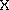2) test (NS 01-Box 3):

 N genotypes # Observed Expected (obs-exp) d2/exp MM p2N (0.539)2(6129) 1787 1781 6 0.020 MN 2pqN (2)(0.539)(0.461)(6129) 3039 3046 -7 0.012 NN q2N (0.461)2(6129) 1303 1302 1 0.000 6129 61292 = 0.032ns
(cf. critical value p.05[1 d.f.] = 3.84)                               ( p >> 0.05)

Use one degree of freedom, because although there are three observed classes,
expectations derived from either one of two alleles

HOMEWORK: S&R Table 3.1 & Eqn 3.2 are wrong: explain the error, correct the calculation
See notes on Chi-Square calculations for some hints

But (you ask) won't "expected" always more or less equal "observed",
cuz that's where "expected" comes from?

Consider artificial data set : MN blood types

 MM MN NN Sum f(M) f(N) Diné 305 52 4 361 0.917 0.083 Koori 22 216 492 730 0.176 0.824 Combined 327 268 496 1091 0.423 0.577

Homework: show
Diné & Koori populations separately exhibit HWP

Chi-square test on combined data:
 Obs Exp d=(O-E) d2/Exp MM 327 195 132 89.35 MN 268 532 -264 131.01 NN 496 364 132 47.872 = 268.23***
(p << 0.001)

*=> A mixture of populations, each of which shows HWP,
will not show expected HWP
if allele frequencies differ in the separate populations.

Wahlund Effect: artificial mixture of populations deficient in heterozygotes [NS 01-02]
(Relate this to F statistics and population structure, later on)

Advanced topics in allele / phenotype frequencies:
Estimating & testing phenotype proportions, with multiple alleles & dominance
Ex. ABO blood group system

Three alleles (A, B, O) produce
six
genotypes (AA, AO, BB, BO, AB, OO) with
four
phenotypes ("A", "B", "AB" "O")
A & B dominant to O; "A" = AA + AO; "B" = BB + BO
A & B" co-dominant as "AB"

Challenge: Cannot obtain exact algebraic solution for four phenotypes from three variables
Therefore use Likelihood method with correction
Ex.: Best a priori likelihood estimate of f(O) is observed[f("O")]

Data from Aka (Mbenga) (Central African Republic) (Cavalli-Sforza & Bodmer 1971)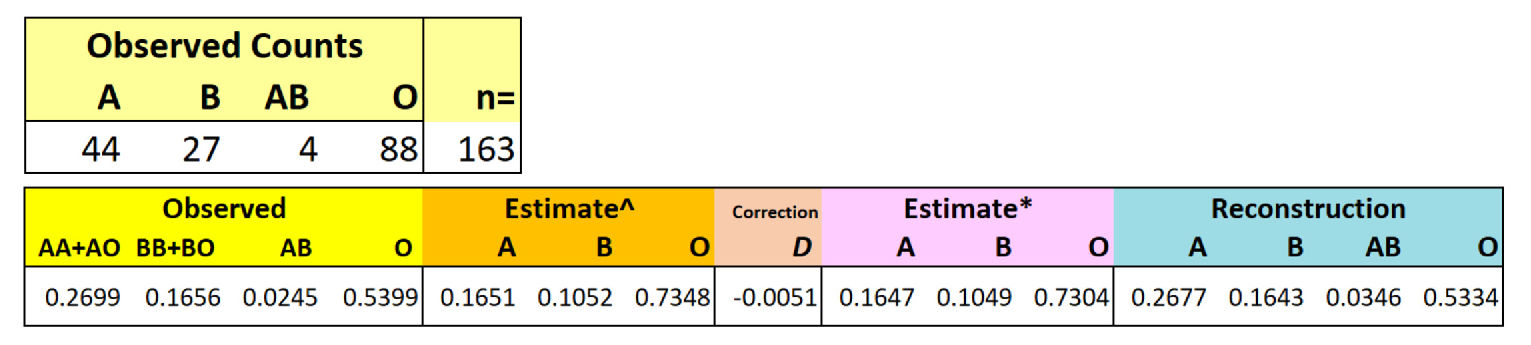HOMEWORK calculate Chi-square for the Observed vs Reconstructed counts

Evolutionary Genetics:
modification of Hardy-Weinberg conditions

Hardy-Weinberg Proportions offer 'null hypothesis':
Consequences of other genetic / evolutionary phenomena?

Five major, interacting factors:

1. Natural selection
Change of allele frequencies (q) [read 'delta q']
occurs due to differential effects of alleles on 'fitness'
Consequences depend on dominance of fitness
[See hardy-weinberg.m MATLAB laboratory exercise]

Natural Selection is the principle concern of micro-evolutionary theory

2. Mutation
A & A' inter-converted at some rate µ
If µ(AA')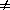µ'(A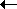A'), net change in frequency

3. Gene flow
Movement of alleles between populations at some rate m
(Im)migration introduces new alleles, changes frequency of existing allele (SR2019 3.12)

4. Statistical sampling error
Chance fluctuations occur in finite populations, especially with small N (SR2019 3.7)
Genetic drift: random change of allele frequencies
over time and (or) space, within and (or) among populations
Modification of N from non-random reproduction: variable sex ratio, offspring number, population size, etc.

5. Population structure
Inbreeding (SR2019 3.2): preferential mating of relatives at some rate F

Inbreeding modifies genotype proportions but not allele frequencies
Assortative Mating
(SR2019 3.4): differential mating of phenotypes and (or) genotypes

Meta-population structure (SR2019 3.8): sub-populations differ wrt total population (F-statistics)

All text material © 2022 by Steven M. Carr